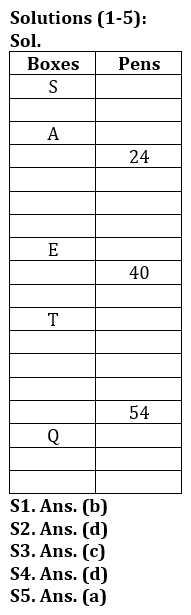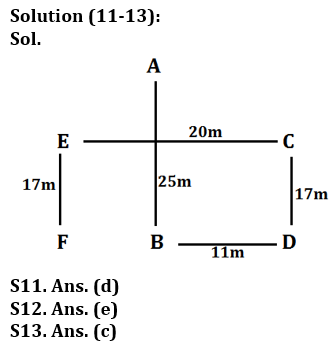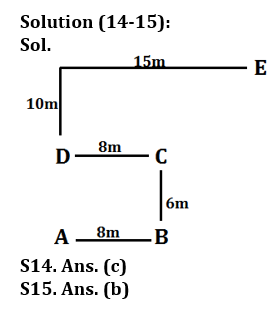# LIC AAO Mains Reasoning Quiz 2023 -12 March

Topic – Practice Set

Directions (1-5): Study the following information carefully and answer the given questions:
A fixed number of boxes are placed on top of each other. Each box contains different pens. Four boxes are placed between A and E. No more than three boxes are placed above A. Three boxes are placed in a box containing E and 24 pens, the box containing 24 pens being placed above the E box. Box S is placed at the topmost position. The number of boxes placed under box A and a box containing 40 pens is half of the number of boxes placed under box E. Four boxes are placed in a box containing 24 pens and a box containing 16 pens. The number of boxes placed in box E and T is one less than the number of boxes placed in T and box which contains 54 pens. Box Q is third from the lowest position. A box containing 54 pens is placed just above Q. The number of boxes placed in T and Q is four times the number of boxes placed in S and A.

Q1. How many boxes are placed in S and T?
(a) Eight
(b) Nine
(c) Six
(d) Five
(e) None

Q2. What will be the position of box M at the top if placed in the middle?
(a) 6
(b) 8
(c) 12
(d) 10
(E) 4

Q3. What is the total number of boxes in this arrangement?
(a) Eighty
(b) Nineteen
(c) Eighteen
(d) Twenty
(e) Cannot be determined

Q4. The number of boxes placed in S and E is equal to the number of boxes placed in Q and ___ boxes.
(a) S
(b) Box containing 24 pens
(c)
(d) Box containing 40 pens
(e) Both (a) and (d)

Q5. What is the product of the number of pens in the fourth box from the top and the number of pens in the fourth box from the bottom?
(a) 1296
(b) 1225
(c) 3025
(d) 788
(e) None of these

Directions (6-10): Answer the questions based on the information given below.
In a particular language,
‘Control beyond world seem’ is coded as ‘R5, H5, H3, R6’.
‘Lock Period Maintain’ is coded as ‘R4, H4, X5, D5’.
‘Would Execute Report’ is coded as ‘K3, H5, A4, K5’.
‘Job failed without’ is coded as ‘L5, R3, D4, R4’.

Q6. What is the code for ‘junior’?
(a) N11
(b) R3
(c) Y7
(d) X4
(e) J1

Q7. What is the code for ‘reviewing’?
(a) R5
(b) H6
(c) U9
(d) X2
(e) D8

Q8. Which of the following words is coded as ‘X3’?
(a) Solid
(b) Meters
(c) Ford
(d) Brain
(e) Wash

Q9. What is the code for ‘COUNT’?
(a) S4
(b) P7
(c) O2
(d) T5
(e) R4

Q10. Which of the following words is coded as ‘H4’?
(a) Solid
(b) Meters
(c) do
(d) Steel
(e) Paper

Directions (11-13): Study the following information carefully and answer the questions given below.
P%Q (10)- P is 12m north of Q
P\$Q (15)- P is 17m south of Q
P#Q (22)- P is 24m east of Q
P&Q (14)- 16m west of PQ
A%B (23), D#B (9), C%D (15), E&C (18), F\$E (15)

Q11. In which direction is C with respect to B?
(a) Ans
(b) West
(c) North-West
(d) North-East
(e) Southwest

Q12. What is the distance between F and D?
(a) 12 m
(b) 14 m
(c) 16 m
(d) 17 m
(e) 20 m

Q13. What is the direction of E with respect to D?
(a) Ans
(b) East
(c) North-West
(d) South-East
(e) None of these

Directions (14-15): Study the following information carefully and answer the given questions.
Rihan starts from point A and walks 8m to reach point B. From point B, he takes a left turn and walks 6m to reach point C, then again takes a left turn and walks 8m to reach point D. From point D he takes two consecutive right turns and walks 10m and 15m respectively to reach point E.

Q14. What is the shortest distance between point A and point C?
(a) 12 m
(b) 16 m
(c) 10 m
(d) 15 m
(e) None of these

Q15. What is the direction of point E with respect to point D?
(a) South-West
(b) North-East
(c) East
(d) North-West
(e) None of these

Solution:Solutions (6-8):
Sol. The coding language used to encode the given words should be decoded in two steps,
Step I: The alphabet is the third letter after the second letter (from the left) of the word.
Second step: The number of consonants in the word is one more.
For example: Control is coded as ‘R6’
Alphabet: O + 3 = R
Number: Number of consonants + 1 = 5 + 1 = 6
So, the code for ‘CUBE’ is X3.
The code for ‘METER’ is ‘H4’
The code for ‘FORD’ is ‘R4’
The code for ‘Brain’ is ‘U4’.
The code for ‘WASH’ is ‘D4’ and so on…

S6. A. (d)